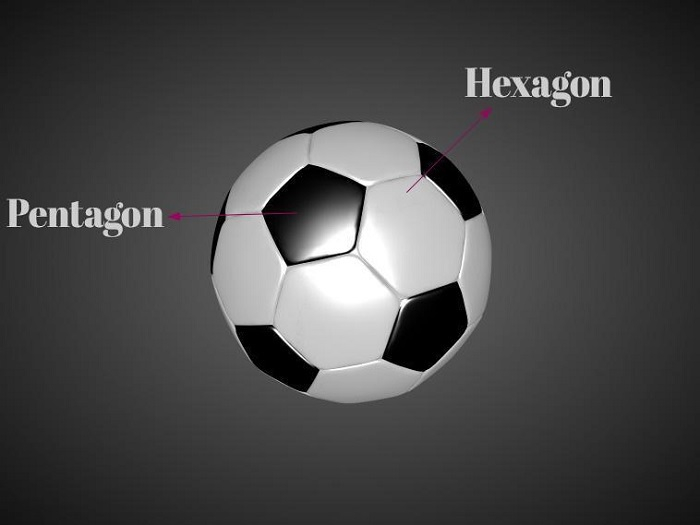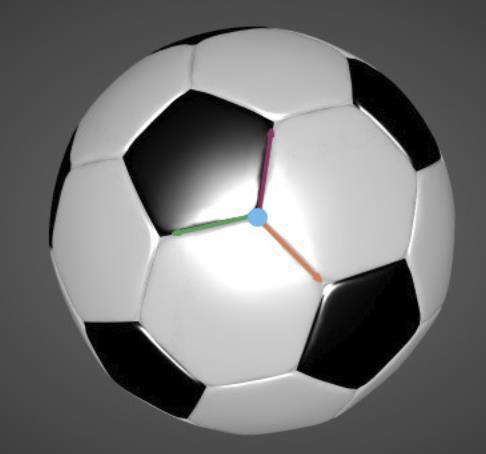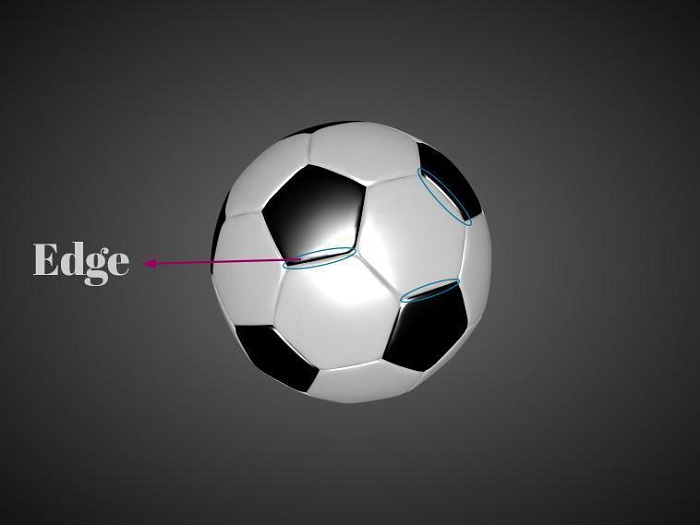# Find the Number of Pentagons and Hexagons on a Football using C++

As we all know, pentagons and hexagons are equally essential parts of football. These shapes fit together like a puzzle for forming a perfectly spherical shape. So here we have a football, in which we have to find the hexagons and pentagons.We will use the Euler characteristic to solve the problem easily. Euler characteristic is a number that works to describe a specific shape or structure of any topological space. So we can use it for calculating the number of Pentagons and Hexagons on the football.

In Euler characteristics −

• chi(S) − Integer for a specific surface S
• F − faces
• G − Graph
• V − Vertices
• E − Edges is embedded in S.

We have,

V - E + F = chi(S)
V - E + F = 2 ……..(A){ for sphere chi(S) = 2 }

Let, number of Pentagon be P and number of Hexagon be H

The number of vertices will be −

Six vertices of the hexagon(6*H) + Five vertices of the pentagon (5*P).

The number of vertices, V = (6*H + 5*P), but we have counted each vertex three times.So Number of vertices,       V = (6*H + 5*P) / 3       ……..(1)

The number of edges will be −

Six edges of the hexagon(6*H) + Five edges of the pentagon (5*P).

The number of edges, E = (6*H + 5*P). However, each edge has been counted twice.Hence Number of edges,      E = (6*H + 5*P) / 2       ……..(2)

Number of faces will be −

Number of hexagon (H) + number of pentagon (P)

F = (H + P)          ……..(3)

Using (1), (2) and (3) in equation (A)

V - E + F = 2

[(6*H + 5*P)/3] - [ (6*H + 5*P)/3 ] + (H + P) = 2

Solving the equation,

P = 12

To calculate the number of hexagons, we know that a hexagon surrounds one pentagon, but we have counted each hexagon thrice for each adjacent pentagon.

Number of Hexagons = 5 * P / 3 = (5 * 12) / 3

H = 20

Finally, we found that a football has −

Count of Hexagons − 20

Count of Pentagons − 12

## Conclusion

So this is how we can find the number of pentagons and hexagons on a football using the Euler characteristic. Pentagons and hexagons play a major role in the creation of the football’s shape. Both of these shapes are enclosed to create the spherical shape of the football. So as you can see in the above solution, we have used different equations to get the required number of pentagons and hexagons on a football.